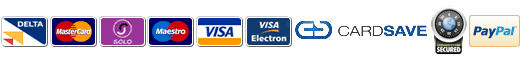## Identifying model year from frame number

To identify the year of a KTM motorcycle look for the 10/11th digit on the frame/vin number.
for example
VBKGSA200 4M 123456 = 2004
VBKGSA200 KM 123456 = 2019

1995=SM 1996=TM 1997=VM 1999=XM 2000=YM
2001=1M 2002=2M 2003=3M 2004=4M 2005=5M 2006=6M 2007=7M 2008=8M 2009=9M
2010=AM 2011=BM 2012=CM 2013=DM 2014=EM 2015=FM 2016=GM 2016=HM 2018=JM 2019=KM
2020=LM 2021=MM 2022=NM

Engine numbers = the first digit will identify the year of manufacture within a decade.
for exanple 5 590 12345 could be 1995, 2005 or 2015 , it can be narrowed down using the next three digits which are the model code. In this case 590 which is a RFS engine made between 2000 and 2007.